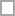# Whole Number Quizzes & Trivia

This is a timed whole number quiz.

Questions: 20  |  Attempts: 218   |  Last updated: Jan 10, 2013
• Sample Question
True/False An even number plus an odd number is always an odd number.This quiz is used as part of an eLearning program. If you can pass it, you don't need to proceed with the rest of the multiplication tutorial.

Questions: 7  |  Attempts: 188   |  Last updated: Jan 3, 2013
• Sample Question
Which of the following symbols represents multiplication?This quiz will test your foundation skills in addition. If you pass it without the use of a calculator, then you're off to a good start.

Questions: 10  |  Attempts: 178   |  Last updated: Jan 3, 2013
• Sample Question
Which of these symbols is used to represent addition?This quiz is used as part of an eLearning program. If you can pass it, you don't need to proceed with the rest of the division tutorial.

Questions: 10  |  Attempts: 134   |  Last updated: Jan 3, 2013
• Sample Question
Which of the following symols represents divison?This quiz will test your foundation skills in subtraction. If you pass it without the use of a calculator, then you're off to a good start.

Questions: 10  |  Attempts: 80   |  Last updated: Jan 3, 2013
• Sample Question
Which of the following symbols represents subtraction?Related

## Whole Number Questions and Answers

• True/False An even number plus an odd number is always an odd number.Whole number question from

• True/False An odd number minus an even number is always an even number.Whole number question from

• 2378 + 1321 =Whole number question from

• Which of the following symbols represents multiplication?Whole number question from

• To multiply means to add up groups of objects. True or False?Whole number question from

• Which of these symbols is used to represent addition?Whole number question from

• What are some other words for addition? (Select all that apply)Whole number question from

• Addition means to put one group of items together with other items and then counting to see how many items there are all together. True of False?Whole number question from

• Which of the following symols represents divison?Whole number question from

• What are some words used in association with division?(tick all that apply)Whole number question from

• To divide means to break a group of items into smaller and equal groups (True or False)Whole number question from